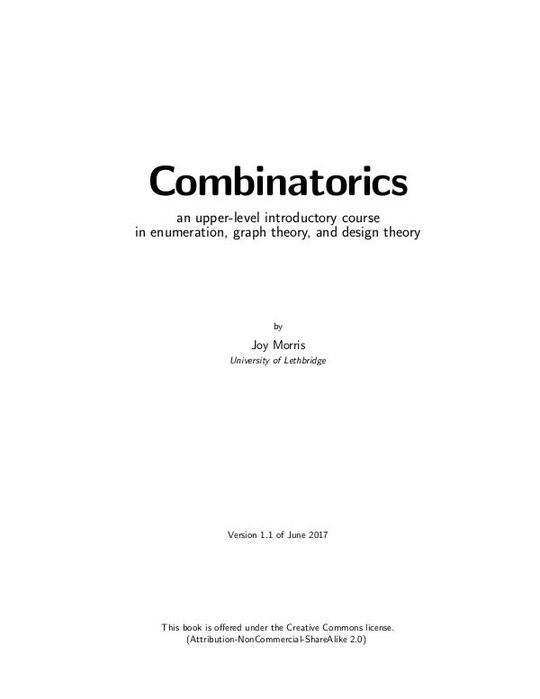OpentextbooksUnknown
Computers & Technology
Combinatorics
Free
The publisher has enabled DRM protection, which means that you need to use the BookFusion iOS, Android or Web app to read this eBook. This eBook cannot be used outside of the BookFusion platform.
Description
Contents
Reviews
Language
Unknown
ISBN
Unknown
Chapter 1. What is Combinatorics?
1A. Enumeration
1B. Graph Theory
1C. Ramsey Theory
1D. Design Theory
1E. Coding Theory
Summary
Part I. Enumeration
Chapter 2. Basic Counting Techniques
2A. The product rule
2B. The sum rule
2C. Putting them together
2D. Summing up
Summary
Chapter 3. Permutations, Combinations, and the Binomial Theorem
3A. Permutations
3B. Combinations
3C. The Binomial Theorem
Summary
Chapter 4. Bijections and Combinatorial Proofs
4A. Counting via bijections
4B. Combinatorial proofs
Summary
Chapter 5. Counting with Repetitions
5A. Unlimited repetition
5B. Sorting a set that contains repetition
Summary
Chapter 6. Induction and Recursion
6A. Recursively-defined sequences
6B. Basic induction
Summary
Chapter 7. Generating Functions
7A. What is a generating function?
7B. The Generalised Binomial Theorem
7C. Using generating functions to count things
Summary
Chapter 8. Generating Functions and Recursion
8A. Partial fractions
8B. Factoring polynomials
8C. Using generating functions to solve recursively-defined sequences
Summary
Chapter 9. Some Important Recursively-Defined Sequences
9A. Derangements
9B. Catalan numbers
9C. Bell numbers and exponential generating functions
Summary
Chapter 10. Other Basic Counting Techniques
10A. The Pigeonhole Principle
10B. Inclusion-Exclusion
Summary
Part II. Graph Theory
Chapter 11. Basics of Graph Theory
11A. Background
11B. Basic definitions, terminology, and notation
11C. Deletion, complete graphs, and the Handshaking Lemma
11D. Graph isomorphisms
Summary
Chapter 12. Moving through graphs
12A. Directed graphs
12B. Walks and connectedness
12C. Paths and cycles
12D. Trees
Summary
Chapter 13. Euler and Hamilton
13A. Euler tours and trails
13B. Hamilton paths and cycles
Summary
Chapter 14. Graph Colouring
14A. Edge colouring
14B. Ramsey Theory
14C. Vertex colouring
Summary
Chapter 15. Planar graphs
15A. Planar graphs
15B. Euler's Formula
15C. Map colouring
Summary
Part III. Design Theory
Chapter 16. Latin squares
16A. Latin squares and Sudokus
16B. Mutually orthogonal Latin squares (MOLS)
16C. Systems of distinct representatives
Summary
Chapter 17. Designs
17A. Balanced Incomplete Block Designs (BIBD)
17B. Constructing designs, and existence of designs
17C. Fisher's Inequality
Summary
Chapter 18. More designs
18A. Steiner and Kirkman triple systems
18B. t-designs
18C. Affine planes
18D. Projective planes
Summary
Chapter 19. Designs and Codes
19A. Introduction
19B. Error-correcting codes
19C. Using the generator matrix for encoding
19D. Using the parity-check matrix for decoding
19E. Codes from designs
Summary
Index
List of Notation
Appendix A. Solutions to selected exercises
The book hasn't received reviews yet.